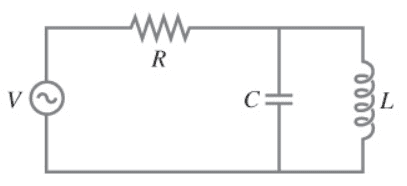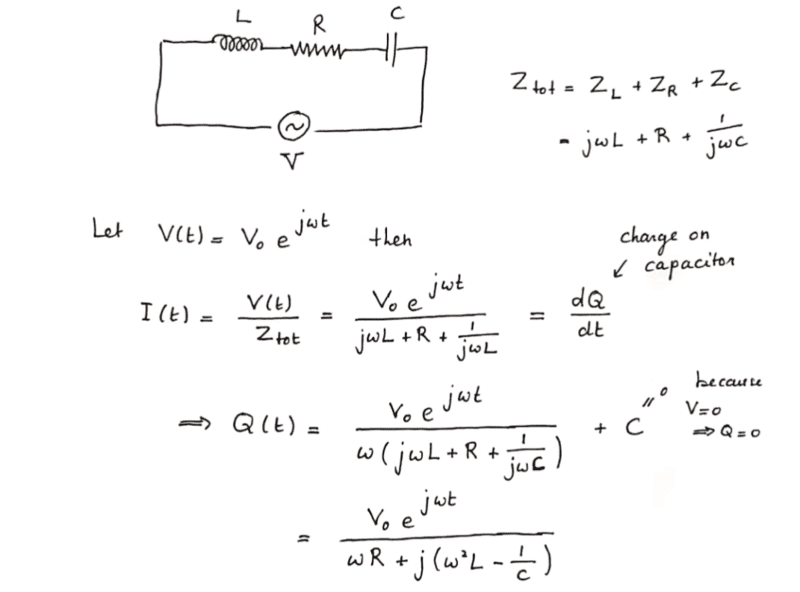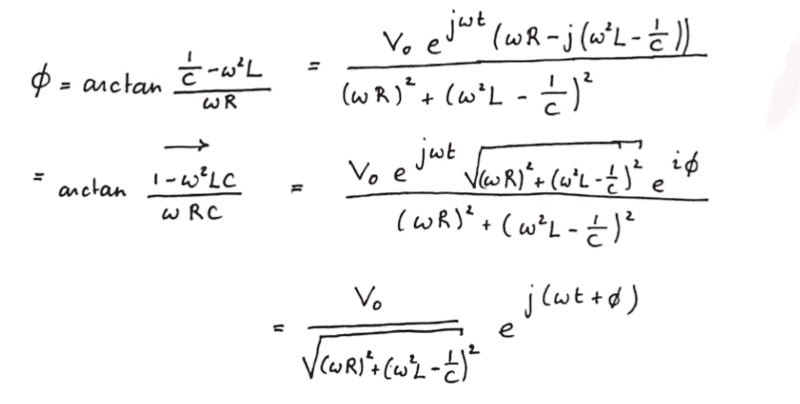# Current flowing through resistor in RLC circuit (C in parallel with L)

• greg_rack
ELI the ICE man... ?)In summary, the conversation revolved around LRC circuits with an AC source and the confusion surrounding the best method to use for solving them. The conversation then delved into applying Ohm's law and computing the equivalent impedance for the circuit. The mistake of inverting the sign in the complex impedance was pointed out, leading to a discrepancy in the calculation of the phase angle. The correct expression for the phase angle was determined to be different by a factor of -1 due to the use of radians instead of degrees and the rotation direction of the phasors.

#### greg_rack

Gold Member
Homework Statement
For the circuit shown, say ##V(t)=V_0sin\omega t##. Calculate the current in the resistor
Relevant Equations
V=ZI
Complex impedances: ##Z_R=R,\ Z_L=j\omega L,\ Z_C=\frac{1}{j\omega C}##Hello guys,
LRC circuits with an AC source are having the best over me... had some confusion in class with respect to which method is best using(phasors diagram, reactances or complex impedances) which I am trying to desperately sort out before my exam; here I will show you my best attempt on this one, which although is missing on something!
Applying Ohm's law ##I(t)=\frac{V(t)}{Z_{eq}}=\frac{e^{j(90-\omega t)}}{Z_{eq}}##, and so the need to compute ##Z##.
Considering the schematics of the circuit, we can just sum up the various impedances accordingly to get to the equivalent one:
$$Z_{eq}=Z_R+(\frac{1}{Z_L}+\frac{1}{Z_L})^{-1}=R+(j(\omega C-\frac{1}{\omega L}))^{-1}= R+j\cdot \frac{1}{\frac{1}{\omega L}-\omega C}$$
Substituting the result above and manipulating the equation:
$$I(t)=\frac{V_0}{\sqrt{R^2+\frac{1}{\frac{1}{\omega L}-\omega C}^2}}\cdot \frac{e^{j(90-\omega t)}}{e^{j\phi}}=\frac{V_0}{\sqrt{R^2+\frac{1}{\frac{1}{\omega L}-\omega C}^2}}\cdot e^{j(90-\omega t-\phi)}=\frac{V_0}{\sqrt{R^2+\frac{1}{\frac{1}{\omega L}-\omega C}^2}}\cdot sin(\omega t +\phi)$$
All we are left with is, at this point, to compute the phase angle ##\phi## which originated from taking the module of Z; for this, what I would do is: ##tan\phi = \frac{Im(Z)}{Re(Z)}=\frac{L}{RC(\frac{1}{\omega C} - \omega L)}## and then write the phase angle as the arctangent of such expression.
The correct result for ##\phi## is, though, ##\phi=atan\frac{L}{RC(-\frac{1}{\omega C} + \omega L)}##, which is what I get but multiplied by '-1'.
This really bothers me, because I am pretty sure to have not made any calculations mistakes and signs are a problem I've been getting quite often when dealing with such problems, which are still somewhat confused in my mind... this makes me think I am missing out on something more important wrt to AC circuits

•Delta2
greg_rack said:
Homework Statement:: For the circuit shown, say ##V(t)=V_0sin\omega t##. Calculate the current in the resistor
Relevant Equations:: V=ZI
Complex impedances: ##Z_R=R,\ Z_L=j\omega L,\ Z_C=\frac{1}{j\omega C}##

View attachment 302704
Hello guys,
LRC circuits with an AC source are having the best over me... had some confusion in class with respect to which method is best using(phasors diagram, reactances or complex impedances) which I am trying to desperately sort out before my exam; here I will show you my best attempt on this one, which although is missing on something!
Applying Ohm's law ##I(t)=\frac{V(t)}{Z_{eq}}=\frac{e^{j(90-\omega t)}}{Z_{eq}}##, and so the need to compute ##Z##.
Considering the schematics of the circuit, we can just sum up the various impedances accordingly to get to the equivalent one:
$$Z_{eq}=Z_R+(\frac{1}{Z_L}+\frac{1}{Z_L})^{-1}=R+(j(\omega C-\frac{1}{\omega L}))^{-1}= R+j\cdot \frac{1}{\frac{1}{\omega L}-\omega C}$$
The mistake is here, you write the term inside the (-1) power as inverse but you also inverse the sign, which is the mistake. So the correct is $$Z=R+j\cdot \frac{1}{\omega C-\frac{1}{\omega L}}$$

It is $$X^{-1}=\frac{1}{X}$$ and NOT $$X^{-1}=\frac{1}{-X}$$.

EDIT:AARGH I blundered badly here, forgot that the imaginary unit is inside the negative power. Well I agree with your result, there must be a mistake in the answer key.

Last edited:
•alan123hk
If you want help determining why your calculation for the phase angle is different by a factor of -1, you will have to show the details of your calculation.

It's likely that the mistake Delta2 pointed out propagated into your calculation of the phase angle.

Last edited:
First, a comment about units. The Euler relation ##e^{j\theta} = \cos \theta + j \sin \theta## is true only if ##\theta## is expressed in radians (not degrees).

You expressed ##\sin \theta## as the real part of ##e^{j(90-\omega t)}##. This should be written as ##e^{j(\pi/2-\omega t)}##.

But ##\sin \omega t## could also be expressed as the real part of ##e^{j(\omega t - \pi/2)}##.

You can check that this second way of expressing ##\sin \omega t## leads to the correct sign for ##\phi##.

So, now you have a conundrum. ##\sin \omega t## can be expressed as either the real part of ##e^{j(\pi/2-\omega t)}## or as the real part of ##e^{j(\omega t - \pi/2)}##. Yet, only the second way yields the right answer for ##\phi##. Why?

Hint: When you use the expressions ##Z_L = j \omega L## and ##Z_C = \frac{1}{j\omega C}## for the complex impedances, then the phasors that represent ##V## and ##I## must rotate counterclockwise (not clockwise). Can you see why? (ELI the ICE man might help.)

Which way does the phasor ##e^{j(\pi/2-\omega t)}## rotate?

•berkeman, greg_rack and Delta2
The Electrician said:
It's likely that the mistake Delta2 pointed out propagated into your calculation of the phase angle.
That's what I thought too but there is no mistake after all, it was my blunder.

I think @TSny found out what's going wrong here.

I think it has something to do with that the first derivative of the complex voltage or the complex current has to correspond to multiplication (of the complex voltage or complex current ) by ##j\omega## if we going to use the expressions ##Z_L=j\omega L , Z_C=\frac{1}{j\omega C}## for the complex impedances.
If you want to use the expression ##X=e^{\frac{\pi}{2}-j\omega t}## for the complex voltage or current then you have to use the expressions ##Z_L=-j\omega L,Z_C=-\frac{1}{j\omega C}##, because the first derivative of ##X## with respect to ##t## is ##X'=-j\omega X##.

This whole thing comes from the fact that the differential equations governing RLC circuits contain the first derivative of current or voltage.

•greg_rack
I 'll try to explain it better here cause I admit my previous explanation at post #6 might be a bit confusing.

In a circuit containing only an inductor (you can do yourself for the case of a capacitor) and voltage sinusoidal source, the governing differential equation is $$V=L\frac{dI}{dt}$$. Now if we assume that the complex voltage is ##V=V_0e^{-j\omega t}## (instead of the standard ##V=V_0e^{j\omega t}## then as one can easily see the solution of this ODE for the current ##I## is (by integrating ##\frac{V}{L}## with respect to ##t##), $$I=\frac{V_0}{-j\omega L}e^{-j\omega t}$$ and hence the complex impedance $$Z_L=\frac{V}{I}=-j\omega L$$, so we see how by putting a negative exponential in the expression for complex voltage, lead us to negative complex impedance.

•greg_rack
First, I agree with your calculation for the impedance Z, and it's phase shift Φ. But ##i(t) = v(t)/Z## there is a 180o phase shift in the division operation. The current is 180o out of phase with the phase of the impedance. I think the discrepancy is between the phase of the impedance and the phase of the current. OTOH, I pretty much ignored all of the ##e^{j⋅(stuff)}## it seemed pointless since you started with ##sin(\omega t)## and ended with ##sin(\omega t + \phi)## .

TSny said:
So, now you have a conundrum. ##\sin \omega t## can be expressed as either the real part of ##e^{j(\pi/2-\omega t)}## or as the real part of ##e^{j(\omega t - \pi/2)}##. Yet, only the second way yields the right answer for ##\phi##. Why?
Ok wow, that is indeed a good point, super cool!
Actually, I would have intuitively said that here the rotation should be CCW because an inductor is present in the circuit... but now this argument seems a bit weak. Is it really all there's to it?
And by the way yes, since -whatever the reason- ##\omega t## has to cause a CCW rotation, a minus sign pops out
TSny said:
Which way does the phasor ##e^{j(\pi/2-\omega t)}## rotate?
as the phasor I used would instead cause a CW rotation

In my opinion the whole point here is that if the complex impedance has phase ##\phi## i.e. $$Z=|Z|e^{j\phi}$$ then the complex current has phase ##-\phi## and not ##\phi##, i.e. it is $$I=I_0e^{j\omega t-\phi}$$.

Does the problem asks for the phase of the current, or the phase of the impedance?

@Delta2 @TSny and everyone else, I will put here also another problem for which I had a very similar issue with phase signs, and for which what was pointed out in #4 doesn't seem to apply.(sorry if I have attached the image directly, but I think it'd be much clearer)

So what happened here was that I was tasked with computing the amplitude of Q(t) across the capacitor in a LRC circuit in series. So same as before, calculate Z, start by assuming ##V(t)=V_0e^{j\omega t}##, write down the current as ##I=\frac{V}{Z}=\frac{dQ}{dQ}## and integrate over time to find a charge function ##Q(t)##.
Just carrying on the calculation takes me to the final expression(developed in column), with the argument of the e term being ##j(\omega t+\phi)##; now, same as before, by calculating ##\phi## "normally" we would get to ##\phi=atan(-(actual\ answer))## so what I did above, of course in hindsight, was just making a minus magically appear by writing ##\phi=\frac{-Imz}{Rez}##(don't tell my mathematics professor)... and so the solution for ##\phi## you find above is the actual correct one, but I don't know for which reason!
Hope I was clear, let me know what you guys think!

•Delta2
You have the charge as $$Q(t)=\frac{V_0e^{j\omega t}}{X}$$ where X is a complex quantity. You write X in the so called "polar form" that is $$X=|X|e^{j\phi}$$ where ##\phi=\arctan ...##. Then its simple complex algebra to see that $$Q(t)=\frac{V_0e^{j\omega t}}{|X|e^{j\phi}}=\frac{V_0}{|X|}e^{j\omega t}e^{-j\phi}$$, that is when the ##e^{j\phi}## goes from denominator to the numerator it has to become ##e^{-j\phi}##.

greg_rack said:
Actually, I would have intuitively said that here the rotation should be CCW because an inductor is present in the circuit... but now this argument seems a bit weak. Is it really all there's to it?

Using CCW rotation ##(e^{j\omega t})## for the complex ##V## and ##I## is a convention. You could use CW rotation ##(e^{-j\omega t})##, but then the complex impedances would have to be ##Z_L = -j\omega L## and ##Z_C = j/\omega C##, as @Delta2 pointed out in post #6.

•Delta2
Delta2 said:
that is when the ##e^{j\phi}## goes from denominator to the numerator it has to become ##e^{-j\phi}##.
But there, the ##e^{j\phi}## factor from polar form is at the numerator, not at the denominator... no?

Hm yes I noticed that. Hold on...

I think there is a mistake in the formula for ##Q(t)## it should be $$Q(t)=\frac{V_0e^{j\omega t}}{j\omega (j\omega L+R+\frac{1}{j\omega C})}$$ that is you missed a ##j## in the denominator. When you integrate ##e^{j\omega t}## the result is $$\frac{1}{j\omega}e^{j\omega t}$$

Delta2 said:
I think there is a mistake in the formula for ##Q(t)## it should be $$Q(t)=\frac{V_0e^{j\omega t}}{j\omega (j\omega L+R+\frac{1}{j\omega C})}$$ that is you missed a ##j## in the denominator. When you integrate ##e^{j\omega t}## the result is $$\frac{1}{j\omega}e^{j\omega t}$$
Oh yeah you are right, but this then would lead to a final minus in front of the whole expression for Q(t), right?
Performing all the calculations, after correctly integrating the e term, leads me to:
$$Q(t)=\frac{-V_0}{\sqrt{(\omega R)^2+(\omega ^2L-1/C)^2}}e^{j(\omega t -\phi)}$$
... unless I should have written the current as ##I=-\frac{dQ}{dt}## instead of ##I=\frac{dQ}{dt}## in the first place, so that the final minus cancels outbut why would this be the case?
It is definitely one of the most messed up topics I have encountered thus far in electromagnetism, super convoluted in terms of signs coming from calculus, complex algebra and weird laws... not even intuitive at all! Hope I will get the hang of it over time, and maybe find a consistent method for analysis!

•Delta2
No you shouldn't express the current as the negative of the charge derivative. What's the problem if there is a minus in front of there?

Delta2 said:
No you shouldn't express the current as the negative of the charge derivative. What's the problem if there is a minus in front of there?
Yeah actually, none I would say. It is still a periodic function, so no big deal

•Delta2
greg_rack said:
@Delta2 @TSny and everyone else, I will put here also another problem for which I had a very similar issue with phase signs, and for which what was pointed out in #4 doesn't seem to apply.
View attachment 302730
View attachment 302731(sorry if I have attached the image directly, but I think it'd be much clearer)

So what happened here was that I was tasked with computing the amplitude of Q(t) across the capacitor in a LRC circuit in series. So same as before, calculate Z, start by assuming ##V(t)=V_0e^{j\omega t}##, write down the current as ##I=\frac{V}{Z}=\frac{dQ}{dQ}## and integrate over time to find a charge function ##Q(t)##.
Just carrying on the calculation takes me to the final expression(developed in column), with the argument of the e term being ##j(\omega t+\phi)##; now, same as before, by calculating ##\phi## "normally" we would get to ##\phi=atan(-(actual\ answer))## so what I did above, of course in hindsight, was just making a minus magically appear by writing ##\phi=\frac{-Imz}{Rez}##(don't tell my mathematics professor)... and so the solution for ##\phi## you find above is the actual correct one, but I don't know for which reason!
Hope I was clear, let me know what you guys think!

It looks to me like you did your phase calculation for the complex denominator in the Q(t) expression. The minus sign you added was, again, because of division. Perhaps like this:

For a, b, c ∈ : ##arg( \frac{c}{a + jb} ) = arg( (\frac{c}{a + jb}) (\frac{a-jb}{a-jb}) ) = arg( \frac{c (a-jb)}{a^2 + b^2}) = atan(\frac{-b}{a}) = -atan(\frac{b}{a})##